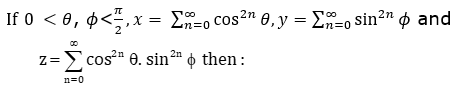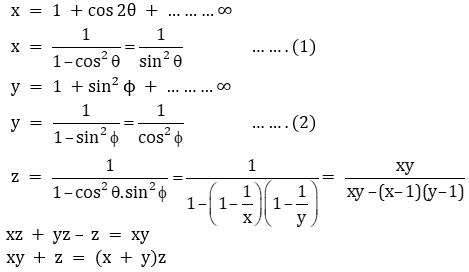# If 0<θ, ϕ < π/2, x = cos2nθa. xyz = 4

b. xy – z = (x + y)z

c. xy + yz + zx = z

d. xy + z = (x+y)z(3)(0)